GeeksforGeeks App
Open AppBrowser
Continue

# Mensuration 2D Formula & Aptitude Questions

Mensuration 2D is an important topic in Quantitative Aptitude, which mainly deals with problems related to the perimeter and area of two-dimensional shapes such as triangles, squares, rectangles, circles, parallelograms, etc. In Quantitative Aptitude, this topic does not have many variations and most of the questions are based on certain fixed formulas. The ability to quickly and accurately calculate the area and perimeter of 2D shapes is an essential skill required for various competitive exams, including aptitude tests.

It is therefore important for candidates to have a good understanding of 2D mensuration concepts and be able to apply them to solve problems

Practice Quiz:

Practice Mensuration 2D Aptitude Quiz Questions

## Triangle

Let the three sides of the triangle be a, b and c.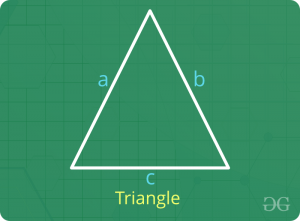• Perimeter = a + b + c
• Area
1. 2s = a + b + c
Area =2. Area = 0.5 x Base x Perpendicular Height

## Rectangle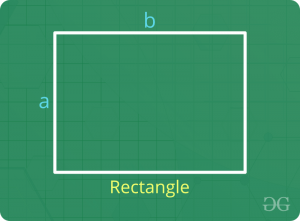• Perimeter = 2 x (length + Breadth)
• Area = Length x Breadth

## Square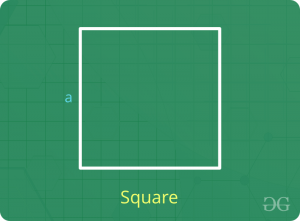• Perimeter = 4 x Side Length
• Area = (Side Length)2 = 0.5 x (Diagonal Length)2

## Parallelogram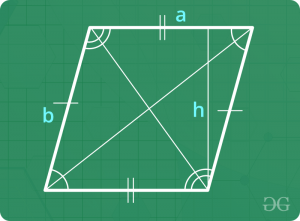• Perimeter = 2 x Sum of adjacent sides
• Area = Base x Perpendicular Height

## Rhombus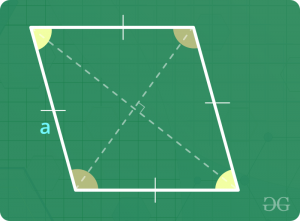• Perimeter = 4 x Side Length
• Area = 0.5 x Product of diagonals

## Trapezium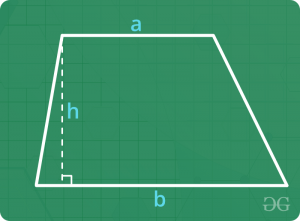• Perimeter = Sum of all sides
• Area = 0.5 x Sum of parallel sides x Perpendicular Height

## Circle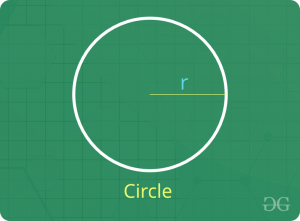• Perimeter = 2 π Radius
• Length of an arc that subtends an angle θ at the center of the circle = (π x Radius x θ) / 180
• Area of a sector that subtends an angle θ at the center of the circle = (π x Radius2 x θ) / 360

## Sample Questions on Mensuration 2D

### Q1: Find the perimeter and area of an isosceles triangle whose equal sides are 5 cm and height is 4 cm.

Solution:

Applying Pythagoras’ theorem,
(Hypotenuse)2 = (Base)2 + (Height)2
=> (5)2 = (0.5 x Base of isosceles triangle)2 + (4)2
=> 0.5 x Base of isosceles triangle = 3
=> Base of isosceles triangle = 6 cm
Therefore, perimeter = sum of all sides = 5 + 5 + 6 = 16 cm
Area of triangle = 0.5 x Base x Height = 0.5 x 6 x 4 = 12 cm2

### Q2: A rectangular piece of dimension 22 cm x 7 cm is used to make a circle of the largest possible radius. Find the area of the circle formed.

Solution:

In questions like this, the diameter of the circle is lesser in length and breadth.
Here, the breadth Diameter of the circle = 7 cm
=> Radius of the circle = 3.5 cm
Therefore, area of the circle = π (Radius)2 = π (3.5)2 = 38.50 cm2

### Q3: A pizza is to be divided into 8 identical pieces. What would be the angle subtended by each piece at the center of the circle?

Solution:

By identical pieces, we mean that area of each piece is the same.
=> Area of each piece = (π x Radius2 x θ) / 360 = (1/8) x Area of circular pizza
=> (π x Radius2 x θ) / 360 = (1/8) x (π x Radius2
=> θ / 360 = 1 / 8
=> θ = 360 / 8 = 45
Therefore, the angle subtended by each piece at the center of the circle = 45 degrees

### Q4: Four cows are tied to each corner of a square field of side 7 cm. The cows are tied with a rope such that each cow grazes the maximum possible field and all the cows graze in equal areas. Find the area of the ungrazed field.

Solution:

For maximum and equal grazing, the length of each rope has to be 3.5 cm.
=> Area grazed by 1 cow = (π x Radius2 x θ) / 360
=> Area grazed by 1 cow = (π x 3.52 x 90) / 360 = (π x 3.52) / 4
=> Area grazed by 4 cows = 4 x [(π x 3.52) / 4] = π x 3.52
=> Area grazed by 4 cows = 38.5 cm2
Now, area of square field = Side2 = 72 = 49 cm2
=> Area ungrazed = Area of field – Area grazed by 4 cows
=> Area ungrazed = 49 – 38.5 = 10.5 cm2

### Q5: Find the area of the largest square that can be inscribed in a circle of radius ‘r’.

Solution:

The largest square that can be inscribed in the circle will have the diameter of the circle as the diagonal of the square.
=> Diagonal of the square = 2 r
=> Side of the square = 2 r / 21/2
=> Side of the square = 21/2
Therefore, area of the square = Side2 = [21/2 r]2 = 2 r2

### Q6: A contractor undertakes the job of fencing a rectangular field of length 100 m and breadth 50 m. The cost of fencing is Rs. 2 per meter and the labor charges are Re. 1 per meter, both paid directly to the contractor. Find the total cost of fencing if 10 % of the amount paid to the contractor is paid as tax to the land authority.

Solution :

Total cost of fencing per meter = Rs. 2 + 1 = Rs. 3
Length of fencing required = Perimeter of the rectangular field = 2 (Length + Breadth)
=> Length of fencing required = 2 x (100 + 50) = 300 meter
=> Amount paid to the contractor = Rs. 3 x 300 = 900
=> Amount paid to the land authority = 10 % of Rs. 900 = Rs. 90
therefore, total cost of fencing = Rs. 900 + 90 = Rs. 990

## Related Resources:

Problems on Mensuration 2D | Set 2

Test your knowledge of Mensuration 2D in Quantitative Aptitude with the quiz linked below, containing numerous practice questions to help you master the topic:-

## Also Practice:

My Personal Notes arrow_drop_up